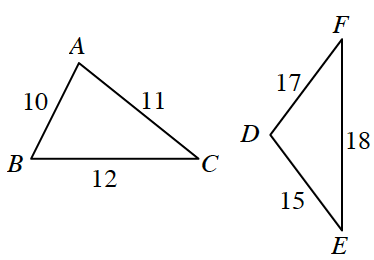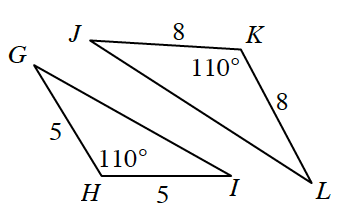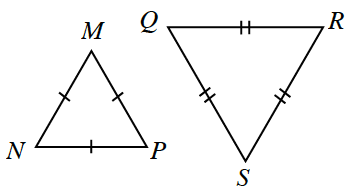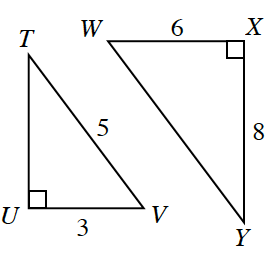### Home > CCG > Chapter 5 > Lesson 5.2.1 > Problem5-58

5-58.

Decide if each pair of triangles below are similar. If they are similar, give a sequence of transformations that justifies your conclusion. If they are not similar, explain how you know.

1.Look at the triangles. Does there seem to be a zoom factor? Do the sides seem to be proportional?

1.How could these triangles be proved similar? Are there any congruent angles? Are there any proportional sides?

$\text{SAS}\sim$ because the angle $110º$ is between both sets of proportional sides.

1.What do the tick marks on the sides of the triangles mean? What type of triangles are they in part (c)?

$\text{SSS}\sim$ because both triangles are equilateral so all sides are proportional.

1.There are different ways that these triangles could be proved similar if you find the missing sides of both triangles. Use the Pythagorean Theorem.